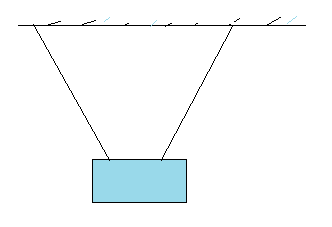Home » Physics » How to solve problems with Body in Static equilibrium

# How to solve problems with Body in Static equilibrium

• Draw a neat diagram of the system.
• Firstly consider the origin of the forces acting on the each object.To do this find out the field forces acting on the each object.Wherever contact in available account the contact force carefully
• Isolate the object being analyzed. Draw a free-body diagram and then show
and label all external forces acting on the object, indicating where those forces are applied.This way there will not any confusion about the force acting on each object.Newtons Third law will help in obtaining the action reaction pair. Do not include forces exerted by the object on its surroundings.(For systems that contain more than one object, draw a separate free-body diagram for each one.) Try to guess the correct direction for each force. If the direction you select leads to a negative force, do not be alarmed; this merely means that the direction of the force is the opposite of what you guessed.
• Establish a convenient coordinate system for the object and find the components of the forces along the two axes. Then apply the first condition for equilibrium. Remember to keep track of the signs of all force components.
• Choose a convenient axis for calculating the net torque on the object. Remember that the choice of origin for the torque equation is arbitrary; therefore, choose an origin that simplifies your calculation as much as possible.
Note that a force that acts along a line passing through the point chosen as the origin gives zero contribution to the torque and thus can be ignored.This site uses Akismet to reduce spam. Learn how your comment data is processed.